Hello friends, I hope you all are doing great. In today’s tutorial, we will have a look at 2nd Year Physics Chapter 12 Solved Questions. I am going to start a new tutorial series about the solution of questions given in the textbook of 2nd-year physics. In this series, we will discuss all the exercise questions of a textbook and find suitable answers.

In this first tutorial of this series, I will explain chapter 12 exercise questions with the detailed and try to make it easy and simple for you. So let’s get started with 2nd Year Physics Chapter 12 Solved Questions.

#### 2nd Year Physics Chapter 12 Solved Questions

• So friends let’s discuss all the questions of chapter 12 one by one with the detailed.

#### Question 12.1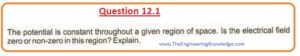• As given that potential is constant through the specific region it means that V1=V2 or we can say that.

V1=V2=V

• Then potential difference will be given as.

ΔV=V2-V1=0

• As we know that

ΔV= -ED

or E= -ΔV/D

E= -0/d=0

• From this, we can conclude that the electric field will also zero.

#### Question 12.2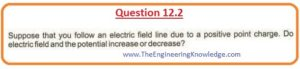• As we know that the formulas of electric field intensity and electric potential are given below.

E=1/4πξ0 . q/r2

V=1/4πξ0 . q/r

•  In above equations, 1/4πξis constant.

E∝ 1/r2

V∝ 1/r

• From these relations, we can observe that if we follow electric field lines than ‘r’ will increases as we are moving away from the point charge.add pic electric field
• The increment in ‘r’ will decreases the electric field intensity and electric potential.

#### Question 12.3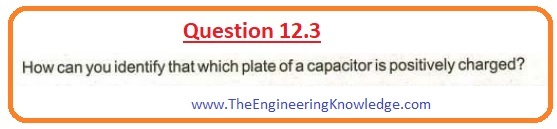• For the detection of the electric field due to any type of charge, we use a positive unit positive charge as a test charge.
• So if we take this charge close to the plate of the capacitor it will bear the force of repulsion if plate is positively charged.

#### Question 12.4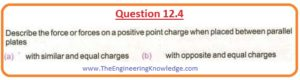(a):

• If we place a positive charge between two positive charged plates then on this charge there will be 2 repulsive forces due to equal charges existing on two plates.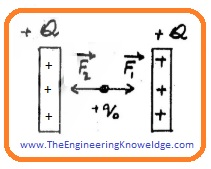• The magnitude of these forces is the same but direction is opposite. So-net force on charge will be zero for the same charged plates.

Fresultant = F1+F2= F-F=0

(b):

Fresultant = F1+F2= F+F=2F

#### Question 12.5• The electric lines of force can not pass or cross each other since the direction and magnitude of electric intensity is one for a given point.
• For the crossing of field lines, there should be more than one value of electric intensity which can be obtained.

#### Question 12.6• The direction of the non-uniform field is always non-linear or curved lines.
• So if we put any point charge ‘q’ of mass ‘m’ in this non-uniform field it will follow the curved lines. Its motion will not be in a straight line.

#### Question 12.7• For this situation, the electric field intensity ‘E’ will be ‘0’. As we know that.

Φe=q/ξ0

• As there is no charge is existing in the blown. so flx will also zero.

q=0, thus  Φe=0

Φe=E.A=0

• In this equation, ‘A’ is not zero but E is zero.charged blownpic

#### Question 12.8• As we know that according to Gauss’s Law.
• Total Flux=1/ξ0 x (total charge in the closed area or surface)

Φe=1/ξ0.(Q)

Φe∝Q

• From this, we can observe that flux intersecting or passing through the closed shaped area is always equal to charged existing in this closed surface.
• Field lines will be continuously moving away from the closed surface having charge in it.

#### Question 12.9• Electrons will move towards high potential or positive charge and move away from less potential due to the same or negative charge.